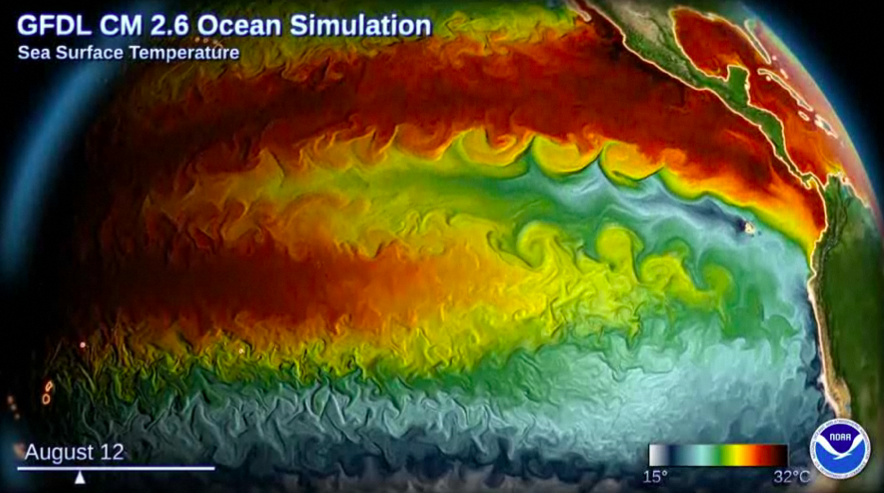# CM2.6 Ocean Model Analysis¶

This notebook shows how to load and analyze ocean data from the GFDL CM2.6 high-resolution climate simulation.Right now the only output available is the 5-day 3D fields of horizontal velocity, temperature, and salinity. We hope to add more going forward.

Thanks to Stephen Griffies for providing the data.

In [ ]:
%matplotlib inline

import numpy as np
import xarray as xr
import matplotlib.pyplot as plt
import holoviews as hv
import intake

hv.extension('bokeh', width=100)


## Create and Connect to Dask Distributed Cluster¶

This will launch a cluster of virtual machines in the cloud.

In [ ]:
from dask.distributed import Client, progress

gateway = Gateway()
cluster = gateway.new_cluster()
cluster.scale(40)
cluster


👆 Don't forget to click this link to get the cluster dashboard

In [ ]:
client = Client(cluster)
client


This data is stored in xarray-zarr format in Google Cloud Storage. This format is optimized for parallel distributed reads from within the cloud environment.

It may take up to a minute to initialize the dataset when you run this cell.

In [ ]:
from intake import open_catalog

cat = open_catalog("https://raw.githubusercontent.com/pangeo-data/pangeo-datastore/master/intake-catalogs/master.yaml"

In [ ]:
# Can also select GFDL_CM2_6_one_percent_ocean
ds


## Visualize Temperature Data with Holoviews and Datashader¶

The cells below show how to interactively explore the dataset.

Warning: it takes ~10-20 seconds to render each image after moving the sliders. Please be patient. There is an open github issue about improving the performance of datashader with this sort of dataset.

In [ ]:
hv_ds = hv.Dataset(ds['temp'])
qm = hv_ds.to(hv.QuadMesh, kdims=["xt_ocean", "yt_ocean"], dynamic=True)

In [ ]:
%%opts QuadMesh [width=800 height=500 colorbar=True] (cmap='magma')
regrid(qm, precompute=True)


## Make an Expensive Calculation¶

Here we make a big reduction by taking the time and zonal mean of the temperature. This demonstrates how the cluster distributes the reads from storage.

In [ ]:
temp_zonal_mean = ds.temp.mean(dim=('time', 'xt_ocean'))
temp_zonal_mean


Depending on the size of your cluster, this next cell will take a while. On a cluster of 40 workers, it took ~12 minutes.

In [ ]:
%time temp_zonal_mean.load()

In [ ]:
fig, ax = plt.subplots(figsize=(16,8))
temp_zonal_mean.plot.contourf(yincrease=False, levels=np.arange(-2,30))
plt.title('Naive Zonal Mean Temperature')

In [ ]: